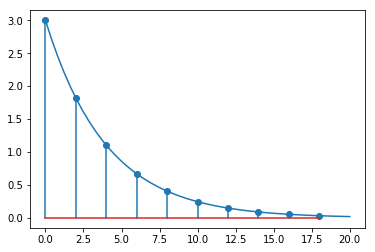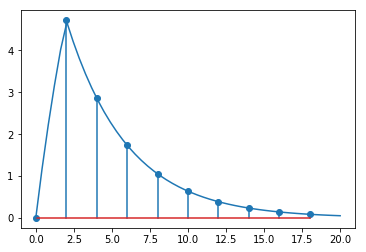:

import sympy
sympy.init_printing()
import matplotlib.pyplot as plt
%matplotlib inline
import tbcontrol
tbcontrol.expectversion('0.1.3')


There is a difference between the $$z$$ transform of an impulse response and the equivalent z transform of a continuous system with a hold element.

Let’s consider the system

$G(s) = \frac{K}{s + r}$
:

s, z, q = sympy.symbols('s, z, q')
K, r, t = sympy.symbols('K, r, t', real=True)
Dt = sympy.Symbol(r'\Delta t', positive=True)

:

G = K/(s + r)


The impulse response of this system is simply the inverse laplace transform:

:

import tbcontrol.symbolic

:

gt = sympy.inverse_laplace_transform(G, s, t)
gt

:

$$K e^{- r t} \theta\left(t\right)$$

The $$z$$ transform of this function of time, sampled at a sampling rate of $$\Delta t$$ can be read off the table as

:

b = sympy.exp(-r*Dt)
Gz = K/(1 - b*z**-1)


Let’s choose values and plot the response.

:

parameters = {K: 3, r: 0.25, Dt: 2}

:

import numpy

:

ts = numpy.linspace(0, 20)

:

terms = 10

:

def plot_discrete(Gz, N, Dt):
ts = [Dt*n for n in range(N)]
values = tbcontrol.symbolic.sampledvalues(Gz.subs(parameters), z, N)
plt.stem(ts, values)

:

def values(expression, ts):
return tbcontrol.symbolic.evaluate_at_times(expression.subs(parameters), t, ts)

:

plt.plot(ts, values(gt, ts))
plot_discrete(Gz, terms, parameters[Dt])But the value in Table 17.1 is

:

a1 = -b
b1 = K/r*(1 - b)
Gz_seborg = (b1 * z**-1)/(1 + a1*z**-1)

:

Gz_seborg

:

$$\frac{K \left(1 - e^{- \Delta t r}\right)}{r z \left(1 - \frac{e^{- \Delta t r}}{z}\right)}$$

That’s clearly not the same as the discrete transform in the datasheet. What is going on?

The values in the table in seborg are the z transform of the transfer function with a hold element!

The z-transform of this combination can be written $$\mathcal{Z}\{H(s)G(s)\}$$. Remember, $$H(s) = \frac{1}{s}(1 - e^{-\Delta t s})$$. Now we can show

\begin{align} \mathcal{Z}\left\{{H(s)G(s)}\right\} &= \mathcal{Z}\left\{\frac{1}{s}(1 - e^{-Ts})G(s)\right\} \\ &= \mathcal{Z}\left\{\underbrace{\frac{G(s)}{s}}_{F(s)}(1 - e^{-Ts})\right\} \\ &= \mathcal{Z}\left\{F(s) - F(s)e^{-Ts}\right\} \\ &= \mathcal{Z}\left\{F(s)\right\} - \mathcal{Z}\left\{F(s)e^{-Ts}\right\} \\ &= F(z) - F(z)z^{-1} \\ &= F(z)(1 - z^{-1}) \end{align}

So the z transform we’re looking for will be $$F(z)(1 - z^{-1})$$ with $$F(z)$$ being the transform on the right of the table of $$\frac{1}{s}G(s)$$.

To remind ourselves,

:

G

:

$$\frac{K}{r + s}$$

So we’re looking for

:

(G/s)

:

$$\frac{K}{s \left(r + s\right)}$$

So we should see the same response if we plot this:

There is an element in the table for

$\frac{a}{s(s + a)}$

which is the same as what we want but multiplied by $$a$$. We should be able to use the associated $$z$$ transform:

:

a = r

:

table_value = (1 - b)*z**-1/((1 - z**-1)*(1 - b*z**-1))

:

Fz = K * table_value / a

:

Fz * (1 - z**-1) == Gz_seborg

:

True

:

response = sympy.inverse_laplace_transform(G/s, s, t)

:

plot_discrete(Gz_seborg, terms, parameters[Dt])
plt.plot(ts, values(response - response.subs(t, t-parameters[Dt]), ts))

:

[<matplotlib.lines.Line2D at 0x1184bacf8>][ ]: